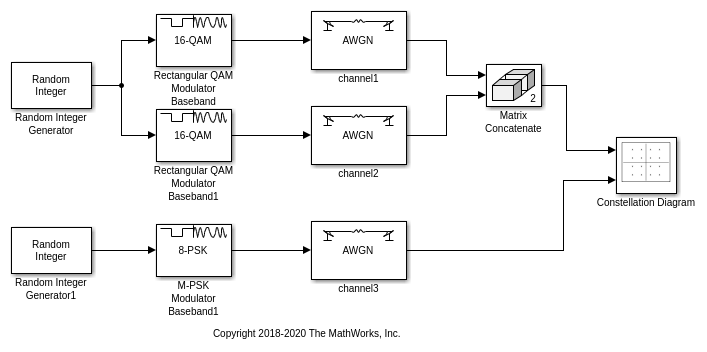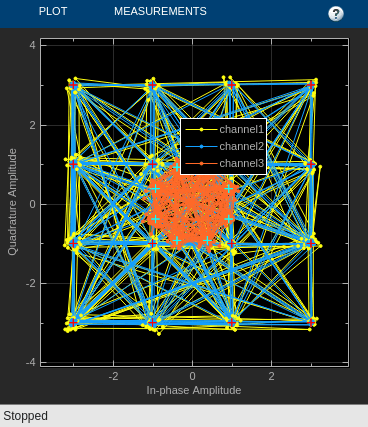Documentation

## View Multi-Input Constellation Diagram

This example shows how to use the Constellation Diagram block to visualize the constellation of multi-input and multichannel modulated signals.

Pass two random 16-QAM signals through the first input port and a random 8-PSK signal through the second input port. One reference constellation can be defined for each input port. Since a single reference constellation is applied for all multichannel signals through one input port, use the Vector Concatenate, Matrix Concatenate block to concatenate multiple signals of the same data type and same reference constellation. In the case where all input signals are single channel input, then they can be directly fed in the Constellation Diagram.

Filter three signals through the AWGN channel and pass them to a Constellation Diagram.

Run Example Model to Explore Its Constellation

To run the model, open the example by entering `open slex_constellation_diagram_multi_channel` at the MATLAB® command prompt.After running the simulation, the constellation diagram shows the noisy constellation points for the input signals. The received data points shown in yellow and blue, represents the two 16-QAM input signals, and the received data points shown in red, represents the 8-PSK signal. The red ‘+’ symbols represent the ideal constellation locations for 16-QAM modulated signals, and the white ‘+’ symbols represent the ideal constellation locations for 8-PSK modulated signal.The trajectory trace is shown for each signal below.Further Exploration

Use the Trace Selection pane to display measurements for the channel of interest. Consider modifying the model to change the current modulation order.